LP Solver Options

This section describes the options recognized by the LP solver. These options can be specified after a forward slash (/) in the SOLVE statement, provided that the LP solver is explicitly specified using a WITH clause.

If the LP solver terminates before reaching an optimal solution, an intermediate solution is available. You can access this solution by using the .sol variable suffix in the OPTMODEL procedure. See the section Suffixes for details.

Solver Options

IIS=numberstring

specifies whether the LP solver attempts to identify a set of constraints and variables that form an irreducible infeasible set (IIS). Table 6.2 describes the valid values of the IIS= option.

Table 6.2: Values for IIS= Option

number

string

Description

0

OFF

Disables IIS detection.

1

ON

Enables IIS detection.

If an IIS is found, information about the infeasibilities can be found in the .status values of the constraints and variables. The default value of this option is OFF. See the section Irreducible Infeasible Set for details about the IIS= option. See Suffixes for details about the .status suffix.

ALGORITHM=option
SOLVER=option
SOL=option

specifies one of the following LP solvers:

Option

Description

PRIMAL (PS)

Uses primal simplex solver.

DUAL (DS)

Uses dual simplex solver.

NETWORK (NS)

Uses network simplex solver.

INTERIORPOINT (IP)

Uses interior point solver.

CONCURRENT (CON) (experimental)

Uses several different algorithms in parallel.

The valid abbreviated value for each option is indicated in parentheses. By default, the dual simplex solver is used.

ALGORITHM2=option
SOLVER2=option

specifies one of the following LP solvers if ALGORITHM=NS:

Option

Description

PRIMAL (PS)

Uses primal simplex solver (after network simplex).

DUAL (DS)

Uses dual simplex solver (after network simplex).

The valid abbreviated value for each option is indicated in parentheses. By default, the LP solver decides which algorithm is best to use after calling the network simplex solver on the extracted network.

Presolve Options

PRESOLVER=number | string

specifies one of the following presolve options:

number

string

Description

0

NONE

Disables presolver.

–1

AUTOMATIC

Applies presolver by using default setting.

1

BASIC

Performs basic presolve like removing empty rows,
columns, and fixed variables.

2

MODERATE

Performs basic presolve and apply other inexpensive
presolve techniques.

3

AGGRESSIVE

Performs moderate presolve and apply other
aggressive (but expensive) presolve techniques.

The default option is AUTOMATIC. See the section Presolve for details.

Control Options

FEASTOL=specifies the feasibility tolerance,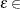[1E–9, 1E–4], for determining the feasibility of a variable. The default value is 1E–6.

LOGFREQ=k
PRINTFREQ=k

specifies that the printing of the solution progress to the iteration log is to occur after every k iterations. The print frequency, k, is an integer between zero and the largest four-byte signed integer, which is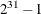.

The value k = 0 disables the printing of the progress of the solution. If the primal or dual simplex algorithms are used, the default value of this option is determined dynamically according to the problem size. If the network simplex algorithm is used, the default value of this option is 10,000. If the interior point algorithm is used, the default value of this option is 1.

LOGLEVEL=number | string
PRINTLEVEL2=number | string

controls the amount of information displayed in the SAS log by the LP solver, from a short description of presolve information and summary to details at each iteration. Table 6.3 describes the valid values for this option.

Table 6.3: Values for LOGLEVEL= Option

number

string

Description

0

NONE

Turns off all solver-related messages to SAS log.

1

BASIC

Displays a solver summary after stopping.

2

MODERATE

Prints a solver summary and an iteration log by using the interval dictated by the LOGFREQ= option.

3

AGGRESSIVE

Prints a detailed solver summary and an iteration log by using the interval dictated by the LOGFREQ= option.

The default value is MODERATE.

MAXITER=k

specifies the maximum number of iterations. The value k can be any integer between one and the largest four-byte signed integer, which is. If you do not specify this option, the procedure does not stop based on the number of iterations performed. For network simplex, this iteration limit corresponds to the solver called after network simplex (either primal or dual simplex).

MAXTIME=t

specifies an upper limit of t units of time for the optimization process, including problem generation time and solution time. The value of the TIMETYPE= option determines the type of units used. If you do not specify the MAXTIME= option, the solver does not stop based on the amount of time elapsed. The value of t can be any positive number; the default value is the positive number that has the largest absolute value that can be represented in your operating environment.

OPTTOL=specifies the optimality tolerance,[1E–9, 1E–4], for declaring optimality. The default value is 1E–6.

TIMETYPE=numberstring

specifies the units of time used by the MAXTIME= option and reported by the PRESOLVE_TIME and SOLUTION_TIME terms in the _OROPTMODEL_ macro variable. Table 6.4 describes the valid values of the TIMETYPE= option.

Table 6.4: Values for TIMETYPE= Option

number

string

Description

0

CPU

Specifies units of CPU time.

1

REAL

Specifies units of real time.

The Optimization Statistics table, an output of the OPTMODEL procedure if you specify the PRINTLEVEL=2 option in the PROC OPTMODEL statement, also includes the same time units for Presolver Time and Solver Time. The other times (such as Problem Generation Time) in the Optimization Statistics table are always CPU times.

The default value of the TIMETYPE= option depends on the values of the NTHREADS= and NODES= options in the PERFORMANCE statement of the OPTMODEL procedure. See the section PERFORMANCE Statement in Chapter 4: Shared Concepts and Topics. for more information about the NTHREADS= and NODES= options.

If you specify a value greater than 1 for either the NTHREADS= or NODES= option, the default value of the TIMETYPE= option is REAL. If you specify a value of 1 for both the NTHREADS= and NODES= options, the default value of the TIMETYPE= option is CPU.

Simplex Algorithm Options

BASIS=number | string

specifies the following options for generating an initial basis:

number

string

Description

0

CRASH

Generate an initial basis by using crash
techniques (Maros, 2003). The procedure creates a
triangular basic matrix consisting of both decision
variables and slack variables.

1

SLACK

Generate an initial basis by using all slack variables.

2

WARMSTART

Start the primal and dual simplex solvers with available basis.

The default option for the primal simplex solver is CRASH (0). The default option for the dual simplex solver is SLACK(1). For network simplex, this option has no effect.

PRICETYPE=number | string

specifies one of the following pricing strategies for the primal and dual simplex solvers:

number

string

Description

0

HYBRID

Use hybrid Devex and steepest-edge pricing
strategies. Available for primal simplex solver only.

1

PARTIAL

Use partial pricing strategy. Optionally, you can
specify QUEUESIZE=. Available for primal
simplex solver only.

2

FULL

Use the most negative reduced cost pricing strategy.

3

DEVEX

Use Devex pricing strategy.

4

STEEPESTEDGE

Use steepest-edge pricing strategy.

The default pricing strategy for the primal simplex solver is HYBRID and that for the dual simplex solver is STEEPESTEDGE. For the network simplex solver, this option applies only to the solver specified by the ALGORITHM2= option. See the section Pricing Strategies for the Primal and Dual Simplex Solvers for details.

QUEUESIZE=specifies the queue size,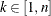, where n is the number of decision variables. This queue is used for finding an entering variable in the simplex iteration. The default value is chosen adaptively based on the number of decision variables. This option is used only when PRICETYPE=PARTIAL.

SCALE=number | string

specifies one of the following scaling options:

number

string

Description

0

NONE

Disable scaling.

–1

AUTOMATIC

Automatically apply scaling procedure if necessary.

The default option is AUTOMATIC.

Interior Point Algorithm Options

CROSSOVER=number | string

specifies whether to convert the interior point solution to a basic simplex solution. The values of this option are:

number

string

Description

0

OFF

Disable crossover.

1

ON

Apply the crossover algorithm to the interior point solution.

If the interior point algorithm terminates with a solution, the crossover algorithm uses the interior point solution to create an initial basic solution. After performing primal fixing and dual fixing, the crossover algorithm calls a simplex algorithm to locate an optimal basic solution. The default value of the CROSSOVER= option is OFF.

STOP_DG=
specifies the desired relative duality gap,
[1E–9, 1E–4]. This is the relative difference between the primal and dual objective function values and is the primary solution quality parameter. The default value is 1E–6. See the section The Interior Point Algorithm for details.

STOP_DI=specifies the maximum allowed relative dual constraints violation,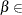[1E–9, 1E–4]. The default value is 1E–6. See the section The Interior Point Algorithm for details.

STOP_PI=specifies the maximum allowed relative bound and primal constraints violation,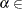[1E–9, 1E–4]. The default value is 1E–6. See the section The Interior Point Algorithm for details.

Decomposition Algorithm Options

The following options are available for the decomposition algorithm in the LP solver. For information about the decomposition algorithm, see Chapter 13: The Decomposition Algorithm.

DECOMP=(options)